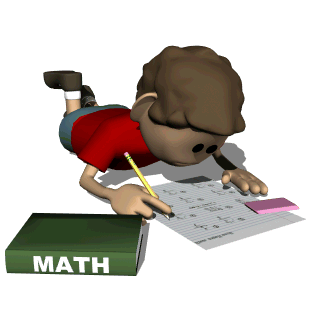•### Eurika Math Parent Homework Tips Handouts:

Second Grade: Overview More learning time should be devoted to working with whole numbers and place value than any other topic.

1. Extend place value understanding of whole number relationships and place value, including grouping in hundreds, tens and ones.

2. Develop competency of strategies for addition and subtraction.

3. Develop understanding of standard units of measure.

(1) Students extend their understanding of place value using the base-ten system. This includes ideas of counting by ones, fives, tens, and hundreds as well as understanding number relationships involving these units, including comparing. Students understand multi-digit numbers through 1000 written in base-ten notation, recognizing that the digits in each place represent amounts of hundreds, tens, or ones.

(2) Students use their understanding of addition to develop fluency with addition and subtraction within 20. They demonstrate understanding of addition and subtraction within 1000 with the use of models. They develop, discuss, and use efficient, accurate, and generalizable methods to compute sums and differences of whole number using base-ten notation, understanding of place value, and the properties of operations. They select and accurately apply methods that are appropriate for the context and the numbers involved to mentally calculate sums and differences.

(3) Students develop understanding for standard units of measure (centimeter and inch), and they use rulers and other measurement tools with the understanding that linear measurement involves an iteration (repetition) of units. They recognize that the smaller the unit, the more iterations they need to cover a given length.

The Standards for Mathematical Practice complement the content standards so that studentsincreasingly engage with the subject matter as they grow in mathematical maturity and expertise throughout the elementary, middle, and high school years.